Sol 1 Using the slope-intercept form,wherewhen, so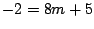and therefore. Therefore the line has equation.

Sol 2 The slope of the line is given by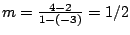, so its equation is given byor.

Sol 3 Since the two points have the same x-coordinate, the line passing through these points is vertical; so its equation is simply.

Sol 4 Solving the equationfor y gives, so its slope is given byand therefore a perpendicular line has slope given by. Using the point-slope form, we get the equation; and simplifying gives.

Sol 5 The perpendicular bisector will pass through the midpoint of the line segment, which is given by. The slope of the line segment is given by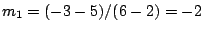, so the slope of the perpendicular bisector will be. Therefore the perpendicular bisector has the equationor.

Sol 6 The line through the two given points has slope, so the line we want will also have slopesince the two lines are parallel. Thus its equation is given by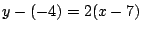or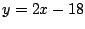.

Sol 7 The tangent line at the pointwill be perpendicular to the line segment from the centerto the point. Since this line segment has slope, the tangent line has the slope. Since the tangent line passes through the point, its equation isor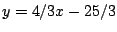.

Sol 8 Multiplying the first equation by 2 gives, and then subtracting the second equation from the first givesor. Then substituting back into the first equation gives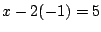so. Therefore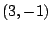is the point of intersection of the lines.